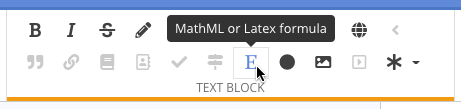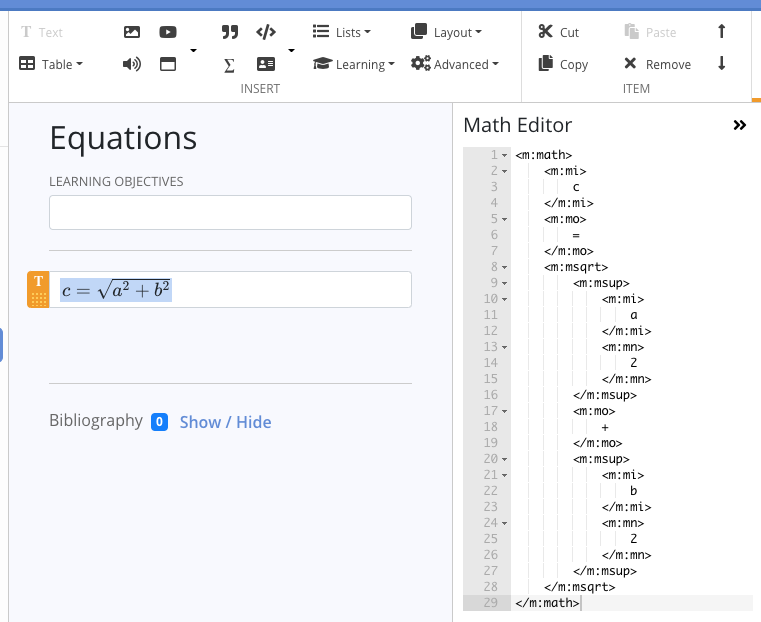Add an equation to the page written in MathML or LaTex.

## MathML

1. #### Place your cursor in the Text field where you want your equation.

2. Click the capital letter E in the Text Block area of the ribbon.3. A MathML equation will be added to the text field. Click on the equation to access its editor in the details panel on the right side of the screen.## LaTex

1. Use these samples for inline or block-level LaTex equations, added directly to the text field. There is not a LaTex editor in the Details panel, like the MathML editor. Use the Page Preview function at the right end of the ribbon to preview your LaTex equation.
• Inline: \( E=mc^2 \)
• Block: \[ E=mc^2 \]

The Echo view:The Page Preview: